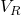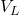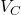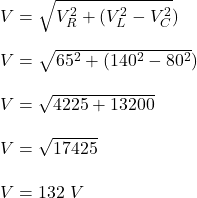A resistor, inductor, and capacitor are connected in series, each with effective (rms) voltage of 65 V, 140 V, and 80 V respectively. What i

Question

A resistor, inductor, and capacitor are connected in series, each with effective (rms) voltage of 65 V, 140 V, and 80 V respectively. What is the value of the effective (rms) voltage of the applied source in the circuit

in progress 0
5 months 2021-08-18T14:33:51+00:00 1 Answers 0 views 0

The value of the effective (rms) voltage of the applied source in the circuit is 132 V

Explanation:

Given;

effective (rms) voltage of the resistor,= 65 V

effective (rms) voltage of the inductor,= 140 V

effective (rms) voltage of the capacitor,= 80 V

Determine the value of the effective (rms) voltage of the applied source in the circuit;Therefore, the value of the effective (rms) voltage of the applied source in the circuit is 132 V.